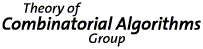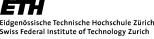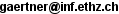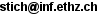## Theory of Combinatorial Algorithms

Prof. Emo Welzl and Prof. Bernd Gärtner

Approximation Algorithms and Semidefinite Programming - course at ETH Zurich# Approximation Algorithms and Semidefinite Programming (252-1426-00L) FS12

## Time & Place

Lecture: Thursday 11:15-12:00 HG E33.3 and Friday 10:15-12:00 CAB G52. The lecturers are:
Bernd Gärtner, CAB G32.2, Tel: 044 632 70 26,.
Jiří Matoušek, CAB G32.2, Tel: 044 632 ?? ??,.
Exercise: Tuesday 16:15-18:00 CAB G15.2. The teaching assistant is:
Sebastian Stich, CAB G39.3, Tel: 044 632 43 29,.

## Course Material

 Date Content Exercises Additional material #1 (23.2.2012) Information about the course; Introduction: Approximating MAX-CUT #2 (24.2.2012) Goemans-Williamson MAX-CUT Approximation via Semidefinite Programming Exercise sheet 1 #3 (1.3.2012) Introduction to Semidefinite Programs #4 (2.3.2012) Complexity of solving Semidefinite Programs; Shannon Capacity Homework 1 #5 (8.3.2012) Shannon Capacity and Theta Number #6 (9.3.2012) Calculating Lovasz Theta Exercise sheet 2 #7 (15.3.2012) The Sandwich Theorem and Perfect Graphs #8 (16.3.2012) Convex Cones and Duality Exercise sheet 3 #9 (22.3.2012) Farkas Lemma #10 (23.3.2012) Cone Programs and Weak Duality Homework 2 #11 (29.3.2012) Duality of Cone Programming #12 (30.3.2012) Optimizing over the Spectrahedron #13 (3.4.2012) Hazan's algorithm (5.4.2012) No lecture Easter Break #14 (19.4.2012) Lower bounds for the Goemans-Williamson MAXCUT algorithm - introduction #15 (20.4.2012) Lower bounds for the Goemans-Williamson MAXCUT algorithm Exercise sheet 4 #16 (26.4.2012) A Hamming graph as a worst-case example for the GW rounding. Proof using eigenvalues for Cayley graphs. #17 (27.4.2012) The Unique Games Conjecture (part 1). Coloring 3-chromatic graphs. Wigderson's trick. Homework 3 #18 (3.5.2012) Properties of the normal distribution. #19 (4.5.2012) The Karger-Motwani-Sudan rounding algorithm. Exercise sheet 5 #20 (10.5.2012) Discrepancy of Set Systems #21 (11.5.2012) Vector Discrepancy and Bansal's Random Walk Algorithm Homework 4 (17.5.2012) No lecture. #22 (18.5.2012) Set Walks. Exercise sheet 6 #23 (24.5.2012) Introduction to Constraint Satisfaction Problems #24 (25.5.2012) Semidefinite Relaxation of Constraint Satisfaction Problems with Triangle Inequalities #25 (31.5.2012) Rounding Via Miniatures #26 (1.6.2012) Rounding Via Miniatures
Topics covered will include:

The Goemans-Williamson MAXCUT algorithm. Semidefinite programming. The Lovasz theta function. Cone programming and duality. Algorithms for semidefinite programming. Advanced applications of semidefinite programming in approximation algorithms.

## Course Description

Over the last fifteen years, semidefinite programming has become an important tool for approximate solutions of hard combinatorial problems. In this lecture, we introduce the foundations of semidefinite programming, we present some of its applications in (but not only in) approximation algorithms, and we show how semidefinite programs can efficiently be solved.

The lecture will follow (parts of) the book "Approximation Algorithms and Semidefinite Programming" by the lecturers (see literature).

## Procedures, exercises, exam

You will receive four small homeworks during the semester. These homeworks are to be solved in written form, but typically you will have two weeks of time to return your solutions/reports, typeset in LaTeX. Your homeworks will be graded and do count towards the final grade: Your three best grades will account for 30% of your final grade. Solving homeworks in teams is not allowed.

There is an oral exam of 30 minutes with 30 minutes of preparation time, which takes place during the examination period. Your final grade consists to 70% of the grade for the exam and to 30% of the grade for the graded homeworks.

You are expected to learn proofs discussed in the lecture, should be able to explain their basic ideas and reproduce more details on demand. You should also be able to give a short presentation on any topic treated throughout the course.

One of the questions given to you during the exam is to solve one of the exercises posed throughout the semester.

Roughly half an hour before the exam you get to know the exercise to be solved and one topic that you will be questioned about in particular, that is, you have 30 minutes preparation time. For this preparation, paper and pencil will be provided. You may not use any other material, like books or notes.

## Further Literature

• Bernd Gärtner and Jiří Matoušek, Approximation Algorithms and Semidefinite Programming, Springer Verlag, 2012.
• David P. Williamson and David B. Shmoys, The Design of Approximation Algorithms, Cambridge University Press, 2011.